Courses

# Important Questions (1 mark): Structure of the Atom

## 25 Questions MCQ Test NCERT Hindi Textbooks (Class 6 to Class 12) | Important Questions (1 mark): Structure of the Atom

Description
This mock test of Important Questions (1 mark): Structure of the Atom for UPSC helps you for every UPSC entrance exam. This contains 25 Multiple Choice Questions for UPSC Important Questions (1 mark): Structure of the Atom (mcq) to study with solutions a complete question bank. The solved questions answers in this Important Questions (1 mark): Structure of the Atom quiz give you a good mix of easy questions and tough questions. UPSC students definitely take this Important Questions (1 mark): Structure of the Atom exercise for a better result in the exam. You can find other Important Questions (1 mark): Structure of the Atom extra questions, long questions & short questions for UPSC on EduRev as well by searching above.
QUESTION: 1

### Which of the following is regarded as a universal particle?

Solution:

Electron is presents in all atoms. So, electron is regarded as universal particle.Electron are distributed in shell around the nucleus.

QUESTION: 2

### The main subatomic particles of nucleus are-

Solution:

The main subatomic particles of nucleus are proton and neutron. Electrons are located in different shells of an atom.

QUESTION: 3

### What is name given to the number of protons in the nucleus of the atom?

Solution:

Number of protons in the nucleus of an atom is equal to atomic number. Sum of number of proton and neutron present in nucleus is called mass number.

QUESTION: 4

The atom which does not contain any neutron in the nucleus is

Solution:

An isotopes of Hydrogen called protium does not contain any neutron. It contain one electron and one proton.

QUESTION: 5

Who discovered anode rays?

Solution:

Anode rays were discovered by E. Goldstein as canal rays. They are positively charged particles.

QUESTION: 6

Match the following with correct response.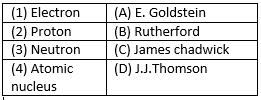Solution:
QUESTION: 7

There are 14 protons and 13 neutrons on the nucleus of an element. What is its mass number?

Solution:

Mass number of an atoms is equal to the sum of number of proton and neutron present in the nucleus of the atom. So, mass number of given element = 14 +13 = 27 u.

QUESTION: 8

“Electrons present in the extra nuclear portion are not stationary rather they revolve around the nucleus at high speed following a circular path” Who said this?

Solution:

In his model of atom, Rutherford stated that electrons present in the extra nuclear portions are not stationary rather they revolve around the nucleus at high speed following circular path.

QUESTION: 9

Match the following with correct response.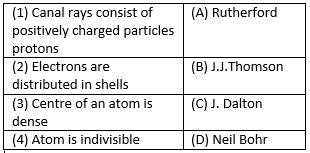Solution:
QUESTION: 10

Nitrogen atom has atomic mass of 14 u and has 7 protons in its nucleus. How many neutrons does it have?

Solution:

Atomic mass of Nitrogen is 14 u and number of protons in nucleus is 7. So, number of neutron = Mass number – number of protons = 14-7 = 7.

QUESTION: 11

Match the following with correct response.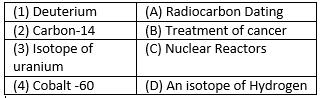Solution:
QUESTION: 12

In an alpha scattering experiment, few alpha particles rebounded because

Solution: In an alpha scattering,few alpha particles rebounded because both alpha particle and nucleus have positive .So,the both of these particles repel each other and nucleus is very dense so alpha particles rebounded.
QUESTION: 13

Match the following with correct response.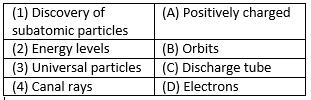Solution:
QUESTION: 14

Atomic number is defined as

Solution:

Atomic number is defined as the number of protons present in the nucleus of an atom.

QUESTION: 15

Isotopes of an element have
A. Same chemical properties
B. Same atomic masses
C. Differeat atomic numbers
D. Atoms of same element

Solution: They have similar chemical properties because isotopes of an element have the same number of electrons as an atom of that element .the electron arrangement is the same owing to same chemical properties how ever they have different number of neutrons which affects the mass and number
QUESTION: 16

How many times is radius of extra nuclear portion more than that of the nucleus of an atom?

Solution:

The radius of extra nuclear portion is more than that of the nucleus of an atom. Nuclear region is small portion of atom as compared to whole atom.

QUESTION: 17

Match the following with correct response.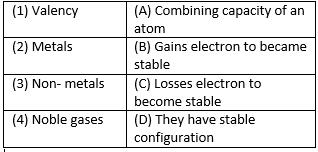Solution:
QUESTION: 18

An atom has a mass number of 23 and atomic number 11. The number of protons are_________.

Solution: The correct option is a. as sodium atom has atomic no 11and atomic no definition itself says that the total no of protons present inside the nucleus of an atom is known as atomic noso no of protons will be 11
QUESTION: 19

How many electrons are present in the species He2+ion?

Solution:

The correct answer is D as its atomic number is 2

it contains 2 electrons and in order to from He2+ ion, it will have to lose its 2 electrons.

QUESTION: 20

Who got the Noble Prize for the work on the structure of atom?

Solution:

Neil Bohr got the noble prize for the work on the structure of atom. He proposed the Bohr model of atom having desecrate shell in which electrons are distributed

QUESTION: 21

Which of the following is not observed by Rutherford in the α-particle scattering experiment?
A. Most of the α-particle rebound after hitting the gold foil
B. Some of the particles deflect by their path
C. Some of the particles did not pass through the gold foil
D. Most of the particles pass straight through the gold foil

Solution:

The correct answer is B as the α-particle scattering experiment gave totally unexpected results.
(i) Most of the fast moving -particles passed straight through the gold foil.
(ii) Some of the -particles were deflected by the foil by small angles.
(iii) Surprisingly one out of every 12000 particles appeared to rebound.

QUESTION: 22

Write number of neutrons in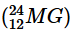Solution:

Magnesium (Mg) contains 12 proton and mass number is 24. Number of neutron is equal to mass number – number of proton present in nucleus. So, number of neutron = 24-12 = 12.

QUESTION: 23

In a species, the number of electrons is more than the number of protons. Predict its nature.

Solution:

Anion contain more electron than proton because anion are formed by receiving electrons from other atoms.

QUESTION: 24

Out of L and M shells which is near to the nucleus of an atom?

Solution:

The order of shell around the nucleus is in order of K, L, M, N from nucleus to outward. So, L shell is nearer to the nucleus than M shell.

QUESTION: 25

Which of the following is correct about atom?
A. It is the smallest unit of matter
B. Size of atom is very large as compared to the nucleus of the atom
C. In an atom number of protons is equal to number of electrons
D. Atoms are stable

Solution:

The nucleus is made up of protons and, in most cases, neutrons. An atomic nucleus is much, much smaller than an atom. The cloud of electrons that "orbit" the nucleus and define the "size" of an atom is roughly 100,000 times as large as that atom's nucleus.